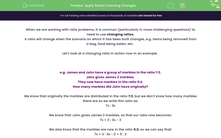# Apply Ratios Involving Changes

In this worksheet, students will solve ratio problems involving changing scenarios using algebra.Key stage:  KS 4

Year:  GCSE

GCSE Subjects:   Maths

GCSE Boards:   AQA, Eduqas, Pearson Edexcel, OCR,

Curriculum topic:   Ratio, Proportion and Rates of Change

Curriculum subtopic:   Ratio, Proportion and Rates of Change Calculations with Ratio

Popular topics:   Percentages worksheets, Ratio worksheets

Difficulty level:#### Worksheet Overview

When we are working with ratio problems, it is common (particularly in more challenging questions) to need to use changing ratios.

A ratio will change when the scenario on which it has been built changes, e.g. items being removed from a bag, food being eaten, etc.

Let's look at a changing ratio in action now in an example.

e.g. James and John have a group of marbles in the ratio 7:3.

John gives James 3 marbles.

They now have marbles in the ratio 5:2.

How many marbles did John have originally?

We know that originally the marbles are distributed in the ratio 7:3, but we don't know how many marbles there are so we write this ratio as:

7x : 3x

We know that John gives James 3 marbles, so that our ratio now becomes:

7x + 3 : 3x - 3

We also know that the marbles are now in the ratio 5:2, so we can say that:

7x + 3 : 3x - 3 = 5 : 2

If these two ratios are equal, we can say that the first part of one, divided by the first part of the other, will be equal to the second part of one, divided by the second part of the other:

 7x + 3 5
=
 3x - 3 2

Using the regular laws of algebra, we can multiply both sides as opposites to reach:

2(7x + 3) = 5(3x - 3)

14x + 6 = 15x - 15

x = 21

Returning to our initial statements, we know that John had 7x marbles originally.

If we substitute '21' for x in this equation, we reach:

7 × 21 = 147 marbles

In this activity, we will apply the algebraic method shown above to changing situations to find starting values, unknown variables or the changing ratio.

### What is EdPlace?

We're your National Curriculum aligned online education content provider helping each child succeed in English, maths and science from year 1 to GCSE. With an EdPlace account you’ll be able to track and measure progress, helping each child achieve their best. We build confidence and attainment by personalising each child’s learning at a level that suits them.

Get started